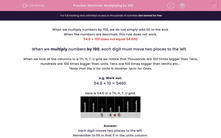# Decimals: Multiplying by 100

In this worksheet, students multiply numbers by 100 by shifting digits two places to the left in a grid.Key stage:  KS 2

Curriculum topic:   Maths and Numerical Reasoning

Curriculum subtopic:   Decimals

Difficulty level:#### Worksheet Overview

When we multiply numbers by 100, we do not simply add 00 to the end.

When the numbers are decimals, this rule does not work.

54.6 × 100 does not equal 54.600

When we multiply numbers by 100, each digit must move two places to the left.

When we look at the columns in a Th, H, T, U grid we notice that Thousands are 100 times bigger than Tens, Hundreds are 100 times bigger than units, Tens are 100 times bigger than tenths etc...

*Note that the U for Units is another term for Ones.

e.g. Work out:

54.6 × 10 = 5460

Here is 54.6 in a Th, H, T, U grid.Each digit moves two places to the left.

Remember to fill in that 0 in the units column.

So  54.6 × 100 = 5460

### What is EdPlace?

We're your National Curriculum aligned online education content provider helping each child succeed in English, maths and science from year 1 to GCSE. With an EdPlace account you’ll be able to track and measure progress, helping each child achieve their best. We build confidence and attainment by personalising each child’s learning at a level that suits them.

Get started••••